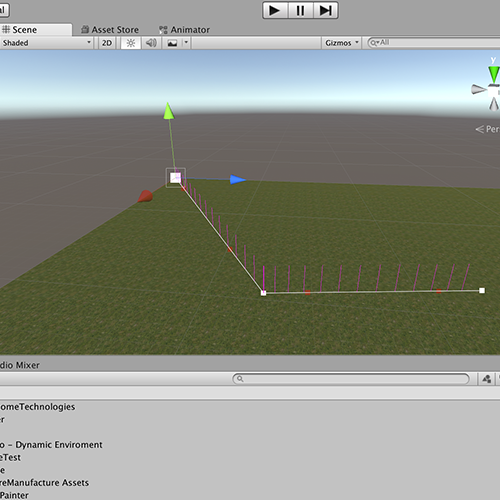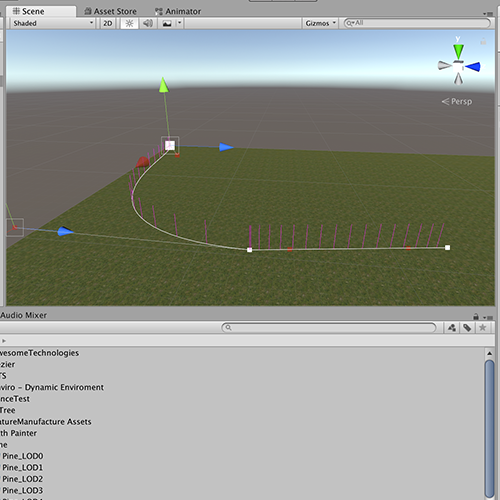# Subdivide Bezier Curves

hi, i want to achieve that I can subdivide a Bezier Curve with a given distance. Now it works if the Bezier is a straight line, but if I change a Controll-Point so the Bezier get’s curved, the Distance will be off what I wanted to achieve! => see pictures at the End of Post

How I get the Subdivision is:

`````` float t = Distance between subdividedParts / bezier length;
//A,B,C,D = ControllPoints of Bezier
GetPoint(A,B,C,D,t);

//GetPoint equation:
public static Vector3 GetPoint (Vector3 p0, Vector3 p1, Vector3 p2, Vector3 p3, float t) {
t = Mathf.Clamp01(t);
float OneMinusT = 1f - t;
return
OneMinusT * OneMinusT * OneMinusT * p0 +
3f * OneMinusT * OneMinusT * t * p1 +
3f * OneMinusT * t * t * p2 +
t * t * t * p3;
}
``````

## I hope enough Information is provided. DanHere is some code for cubic Beziers:

``````void Subdivide(
Vector3 a0, Vector3 a1, Vector3 a2, Vector3 a3, float t,
out Vector3[] firstPart, out Vector3[] secondPart
) {
var b0 = Vector3.Lerp(a0, a1, t); // Same as evaluating a Bezier
var b1 = Vector3.Lerp(a1, a2, t);
var b2 = Vector3.Lerp(a2, a3, t);

var c0 = Vector3.Lerp(b0, b1, t);
var c1 = Vector3.Lerp(b1, b2, t);

var d0 = Vector3.Lerp(c0, c1, t); // This would be the interpolated point

firstPart = new Vector3[] { a0, b0, c0, d0 }; // first point of each step
secondPart = new Vector3[] { a3, b2, c1, d0 }; // last point of each step
}
``````

EDIT:
Ah, now I understand what you meant (I’ll leave the previous answer because it might be helpful for someone else). Unfortunately I think this might be quite a difficult problem. It’s surely possible but it probably involves some nasty integrals. Depending on your requirements, an approximate solution might be enough. Here is one, it’s a bit ugly but it kinda works. It takes any curve (could be Bezier), the number of subdivisions you want to perform and a value that determines how accurate the approximation will be. It returns an array of values such that evaluating the curve with those values yields evenly spaced points.

``````float[] Subdivide(System.Func<float, Vector3> curve, int divisionCount, int approximationSteps) {
// Estimate distances along the curve in regular intervals of t
// by approximating the curve with a polyline
float stepSize = 1f / approximationSteps;
float[] distances = new float[approximationSteps + 1];

Vector3 last = curve(0);
Vector3 current;

for (int i = 1; i < distances.Length; i++) {
current = curve(i * stepSize);
distances *= distances[i - 1] + Vector3.Distance(last, current);*
``````

last = current;
}

// Walk through distance array to find the t for each subdivision
float sectionLength = distances[distances.Length - 1] / (divisionCount + 1);
float[] divisions = new float[divisionCount];

int tInt = 0;
float tFrac;

for (int i = 0; i < divisionCount; i++) {
float target = (i + 1) * sectionLength; // Where the next subdivision should be
while (distances[tInt] < target) tInt++; // Skip until target is exceeded
tFrac = Mathf.InverseLerp(distances[tInt - 1], distances[tInt], target);
divisions = (tInt - 1 + tFrac) / approximationSteps;
}

return divisions;
}
Here is how it could be used:
var divisions = Subdivide(t => Bezier(a, b, c, d, t), 10, 100);
foreach (var t in divisions) {
var p = Bezier(a, b, c, d, t);
Gizmos.DrawLine(p + Vector3.down * 0.1f, p + Vector3.up * 0.1f);
}
If you just need it to look right, this should be accurate enough:
[132784-beziersubdivide.png|132784]_
_
: https://pages.mtu.edu/~shene/COURSES/cs3621/NOTES/spline/Bezier/bezier-sub.html*_
_*

Ok, based on your new image and explanation I think we finally understand what’s your goal. However it’s not that easy to get it working for every kind of cubic bezier curve. The main issue is that certain points can have more than one point that is on the perimeter.

Bezier curves aren’t linear in it’s nature which makes it quite difficult to get some uniform pattern analytically. The best approach is to do some sort Newton’s method to approach the desired point. So you would just nudge forward along the curve and measure the distance between your last point and the current position. Once distance is too large you would decrease the step size and nudge back until you are again below the target distance. Repeat to get as close as you want to your target distance.

One problem is that if your curve has a small sharp turn and your step size is too large that you jump over a possible solution and pick the next intersection instead. If that’s not an issue this is probably the best solution. Since the “density” of the curve changes as you walk along the curve it’s best to measure the relative distance after each step to adjust the step size accordingly.

Basically something like this:

``````float targetLength;

// determine worldspace length of a step of 0.001 units
float L = (C(0.001f) - C(0)).magnitude;

// normalized step size to achieve about 1/10 of our target length.
float step = ((targetLength / 10) / L) * 0.001f;

Vector3 last = C(t);
t += step;
while (true)
{
while ((C(t) - last).sqrMagnitude < targetLength *targetLength && t < 1f)
t += step;
step /= 10f;
while ((C(t) - last).sqrMagnitude > targetLength *targetLength && t > 0)
t -= step;
step /= 10f;
if (Mathf.Abs((C(t) - last).sqrMagnitude - targetLength *targetLength) < 0.0001f || t<0 || t>1)
break;
}
Vector3 next = C(t);
``````

This is untested and should be seen as a pseudo script example.

So the final code works now with connected beziers. thanks a lot, to @Bunny83 (Moved from Comment to Answer, due to. readability)

Structs:

``````	public struct SimpsonVec{
[SerializeField] 	public Vector3 A;
[SerializeField] 	public Vector3 B;
[SerializeField] 	public Vector3 C;
[SerializeField] 	public Vector3 D;
}
public struct  BezierPoints
{
[SerializeField] public List<Vector3> bp_vector3;
[SerializeField] public List<Vector3> bp_lastSpawn;
}
``````

Code for a Vector3[] Array Output:

``````public Vector3[] GetAllPoints(float gap,float acc){

SimpsonVector = SV_SETUP_ALL();
BezierPoints bp = new BezierPoints();
bp.bp_vector3 = new List<Vector3>();
bp.bp_lastSpawn = new List<Vector3>();

for(int i = 0; i<points.Length / 3;i++){

Vector3 ls = new Vector3();
if(i == 0){
ls = Bezier.GetPoint(SimpsonVector.A,SimpsonVector.B,SimpsonVector.C,SimpsonVector.D,0);
}if (i > 0){
ls = bp.bp_lastSpawn[i-1];
}
BezierPoints bp_temp = GetSegmentPoints(gap,acc,i,ls);
SimpsonVector_TEMP = SimpsonVector;
}

return bp.bp_vector3.ToArray();
}

BezierPoints GetSegmentPoints (float gap,float acc,int index, Vector3 ls)
{
SimpsonVec sv = SimpsonVector[index];
Vector3 last_spawn = ls;

BezierPoints bp = new BezierPoints();
bp.bp_vector3 = new List<Vector3>();
bp.bp_lastSpawn = new List<Vector3>();

float step = 0.1f;
float t = step;
float lastT = new float();

while (t >= 0 && t <= 1f)
{
while (t < 1f && Vector3.Distance(Bezier.GetPoint(sv.A,sv.B,sv.C,sv.D,t), last_spawn) < gap){
t += step;}
step /= acc;
while (t > lastT && Vector3.Distance(Bezier.GetPoint(sv.A,sv.B,sv.C,sv.D,t), last_spawn) > gap){
t -= step;}
step /= acc;
if (t > 1f || t < lastT){
break;}
if(step < 0.000001f){
last_spawn = Bezier.GetPoint(sv.A,sv.B,sv.C,sv.D,t);
lastT = t;
step = 0.1f;
}
}
return bp;
}
``````

Helper Methods

``````public SimpsonVec SV_Setup(int index){
SimpsonVec sv;
sv.A = points[index];
sv.B = points[index+1];
sv.C = points[index+2];
sv.D = points[index+3];
return sv;

}
public SimpsonVec[] SV_SETUP_ALL(){
SimpsonVec[] sv = new SimpsonVec[points.Length / 3];
for(int i = 0; i<points.Length / 3;i++){
sv <em>= SV_Setup(i*3);</em>
``````
• }*
• return sv;*
}
public Vector3 GetPoint (Vector3 p0, Vector3 p1, Vector3 p2, Vector3 p3, float t) {
• t = Mathf.Clamp01(t);*
• float OneMinusT = 1f - t;*
• return*
_ OneMinusT * OneMinusT * OneMinusT * p0 +_
_ 3f * OneMinusT * OneMinusT * t * p1 +_
_ 3f * OneMinusT * t * t * p2 +_
_ t * t * t * p3;_
}